# Quiz Generation

The new Grading package in Maple 18 features new and improved tools for creating interactive self-grading quizzes for your students.

With the Quiz command, you can generate interactive questions in Maple that ask a question and grade a student's response, making it easy to provide students with the opportunity to practise, explore, and reinforce concepts.

The Grading package also features a robust toolkit of commands that can be used to build interactive applications. For example, say we have created an application that asks students to describe an expression, such as an arbitrary linear, quadratic, or absolute value function, by interactively graphing it. They can enter this graph in several ways, including by specifying particular points, such as the vertex of a parabola or the roots of an equation. The GradePlot command can then be used to grade the plot to a customizable margin of error by comparing the student's graph with the actual graph of the function.

In addition, a new InertForms package makes it possible to capture student responses precisely as entered, without any automatic simplifications, so you can check both the mathematical content and the exact form of a student response.

 >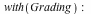### Generating a Quiz

The Quiz command generates a simple interactive tool that displays a question, accepts a response, and grades the response.

The simplest forms of a quiz take two arguments, a question and an answer:

 >What is 1 + 1?It is also possible to generate multiple choice or true false questions:

 >What is 1 + 1?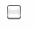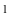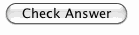You can generate algorithmic questions:

 >Is the following number prime?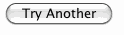You can also grade plotting questions:

 >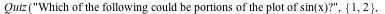Which of the following could be portions of the plot of sin(x)?The GradePlot command allows you to construct representations of simple mathematical functions and then grade them.

In the following examples, an object representing a quadratic function can be created by the QuadraticFunction constructor in one of two ways:

• An expression in a single variable can be provided.
• Alternatively, two points, the first representing the vertex and the second another point on the function, can be given.

The solution as set by the instructor:

 >>A correct answer, if small errors were considered acceptable. In the context of plotted functions, it is more likely that points with a certain amount of error would be provided:

 >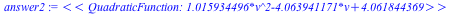>The GradePlot command compares the desired solution to the provided answer and returns a grade of 0 or 1. Since the grading is in the context of plotting, the plot ranges must be provided. Note that the second answer is considered incorrect if a tighter error tolerance is specified.

 >>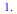>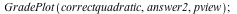>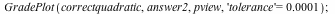>### Working with Constructed Functions

There are several commands available for getting more details on the functions created using the Grading package's constructors. A few examples are shown below:

 >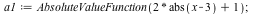>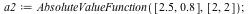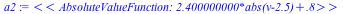To get the expression associated with the object, we can use the GetExpression command:

 >Get the original data used to construct the object using the GetData command:

 >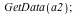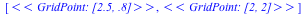Draw an object using the Draw command:

 >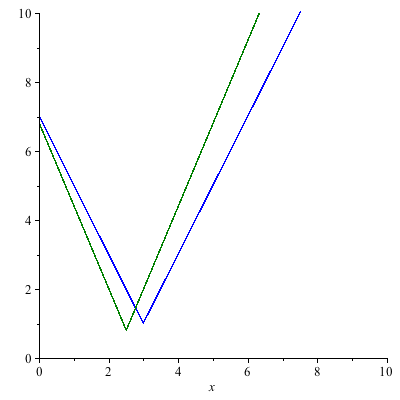The Grading package can be used effectively with embedded components to create grading applications. Normally, the components below would be placed in a single document and the code would be placed in the start-up code region, but it is shown here to illustrate a simple use of the Grading commands.

The example below allows a user to click on two points to draw the given line.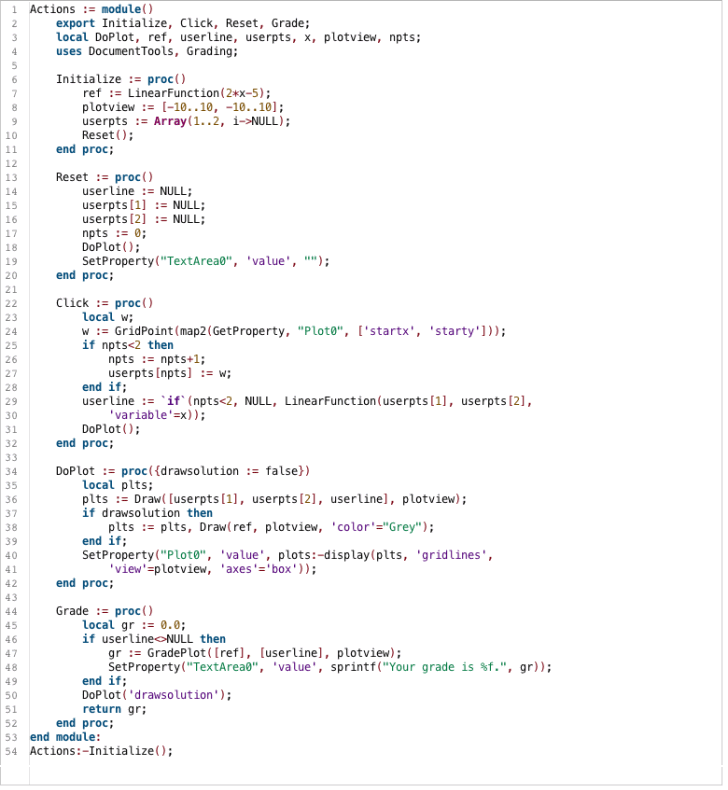### Capturing Exact Student Input

When students use Math Apps, they are sometimes asked to enter a mathematical expression into a math entry box. By default, when Maple is asked what the student entered, Maple applies the same automatic simplification rules as it would if the expression was entered elsewhere in Maple. For example “2/4” would be returned as “1/2”. Most of the time, this is what you want, since you are interested in the mathematical result, not the form it was entered in. But occasionally, you want to know exactly that the student entered, because you want the answer in a particular form.

With the new InertForm package, you can capture the exact input. The InertForm package allows you to extract the contents of a math entry box in a notation that will not evaluate. This notation has the advantage of being an unambiguous representation of a mathematical expression that follows strict rules which make it easy to take apart and compare with the desired response.